# Introduction to Probability and Statistics Chapter 6 The

• Slides: 30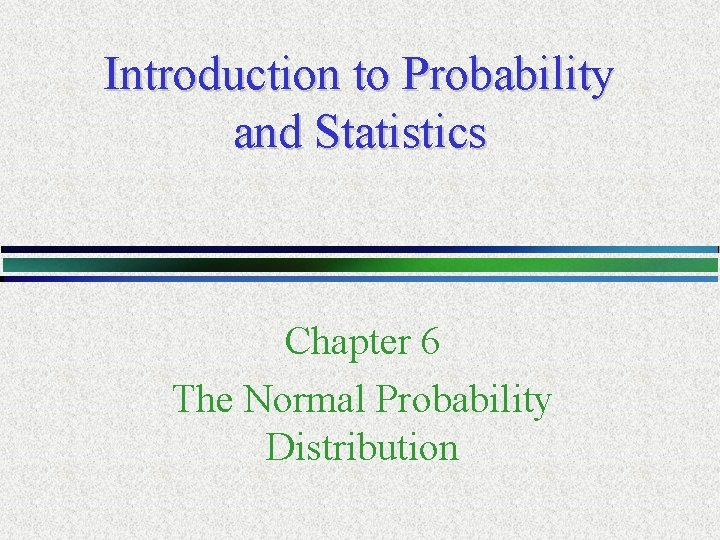Introduction to Probability and Statistics Chapter 6 The Normal Probability DistributionContinuous Random Variables • Continuous random variables can assume infinitely many values corresponding to points on a line interval. • Examples: – Heights, Weights – Lifetime of a particular product – Experimental laboratory error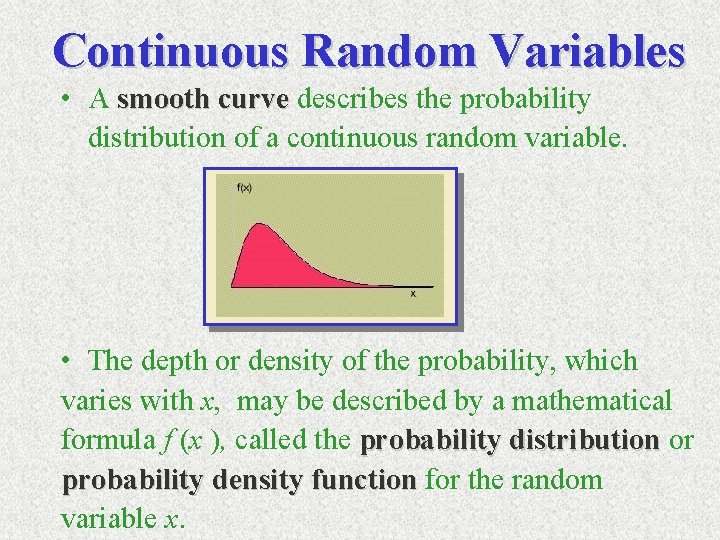Continuous Random Variables • A smooth curve describes the probability distribution of a continuous random variable. • The depth or density of the probability, which varies with x, may be described by a mathematical formula f (x ), called the probability distribution or probability density function for the random variable x.Properties of Continuous Random Variable x • The area under the curve is equal to 1. • P(a < x < b) = area under the curve between a and b. • There is no probability attached to any single value of x. That is, P(x = a) = 0.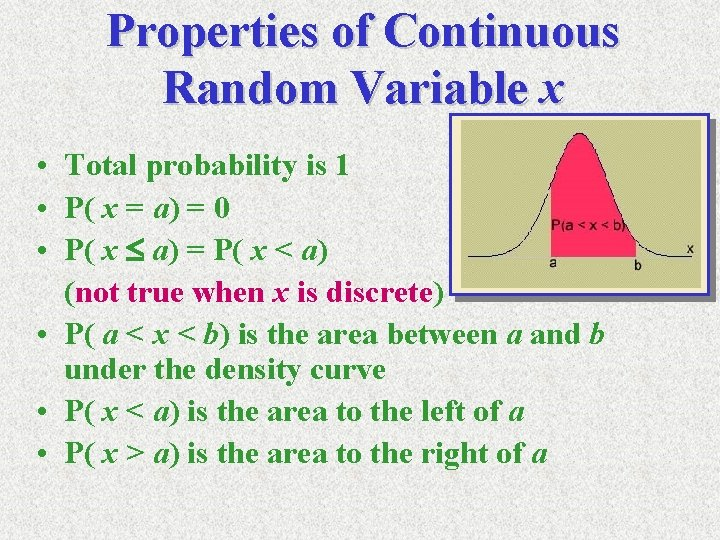Properties of Continuous Random Variable x • Total probability is 1 • P( x = a) = 0 • P( x a) = P( x < a) (not true when x is discrete) • P( a < x < b) is the area between a and b under the density curve • P( x < a) is the area to the left of a • P( x > a) is the area to the right of aContinuous Probability Distributions • There are many different types of continuous random variables a. Uniform; b. Exponential; c. Normal. • We try to pick a model that – Fits the data well – Allows us to make the best possible inferences using the data.Normal Distribution • The formula that generates the normal probability density is: • Standard Normal: m = 0, s = 1.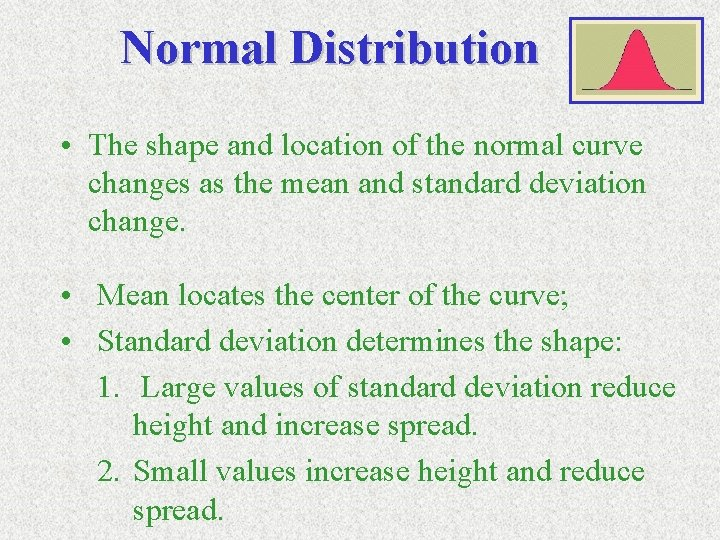Normal Distribution • The shape and location of the normal curve changes as the mean and standard deviation change. • Mean locates the center of the curve; • Standard deviation determines the shape: 1. Large values of standard deviation reduce height and increase spread. 2. Small values increase height and reduce spread.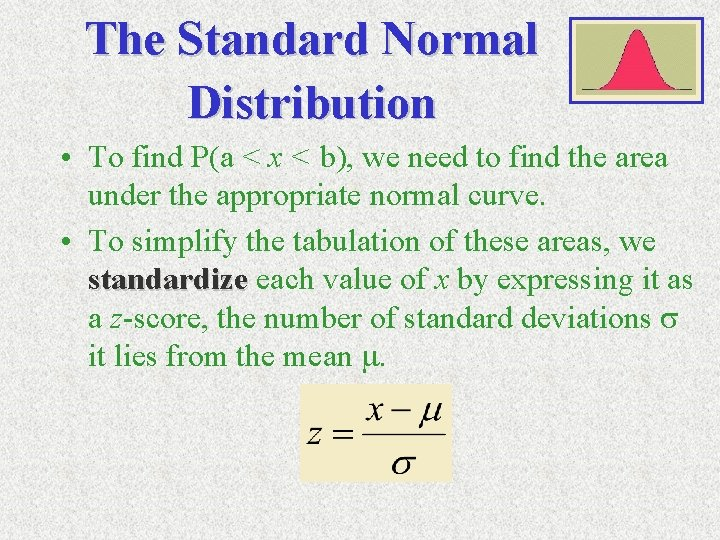The Standard Normal Distribution • To find P(a < x < b), we need to find the area under the appropriate normal curve. • To simplify the tabulation of these areas, we standardize each value of x by expressing it as a z-score, the number of standard deviations s it lies from the mean m.Standard Normal (z) Distribution • • • z has Mean = 0; Standard deviation = 1 Symmetric about z = 0 Total probability Total area under curve is 1; P ( z > 0 ) =. 5 Area to the right of 0 is 0. 5; P ( z < 0 ) =. 5 Area to the left of 0 is 0. 5.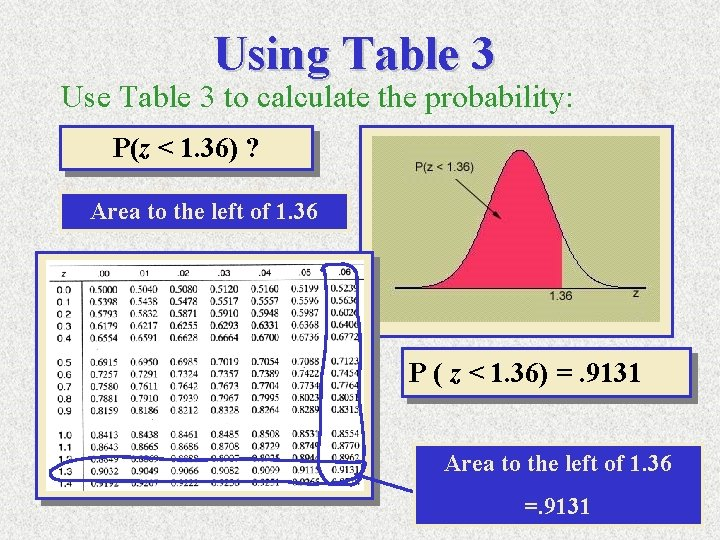Using Table 3 Use Table 3 to calculate the probability: P(z < 1. 36) ? Area to the left of 1. 36 P ( z < 1. 36) =. 9131 Area to the left of 1. 36 =. 9131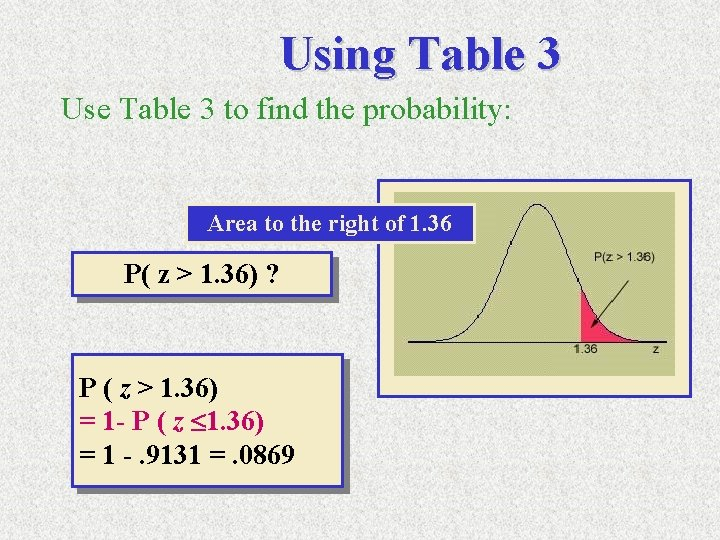Using Table 3 Use Table 3 to find the probability: Area to the right of 1. 36 P( z > 1. 36) ? P ( z > 1. 36) = 1 - P ( z 1. 36) = 1 -. 9131 =. 0869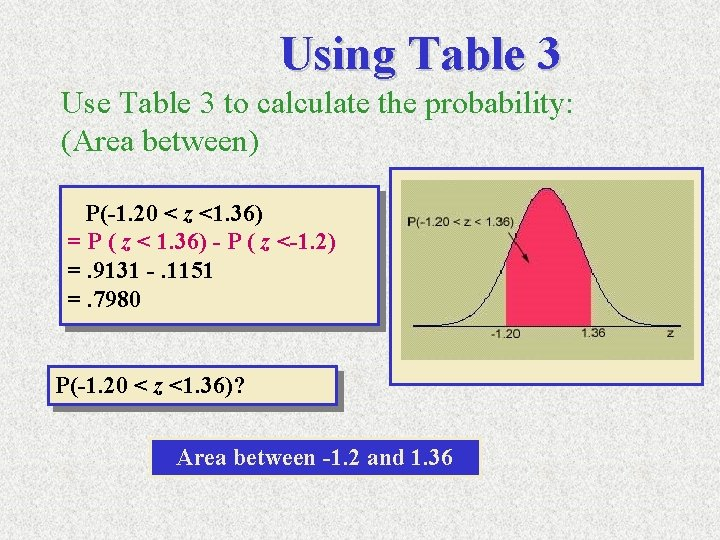Using Table 3 Use Table 3 to calculate the probability: (Area between) P(-1. 20 < z <1. 36) = P ( z < 1. 36) - P ( z <-1. 2) =. 9131 -. 1151 =. 7980 P(-1. 20 < z <1. 36)? Area between -1. 2 and 1. 36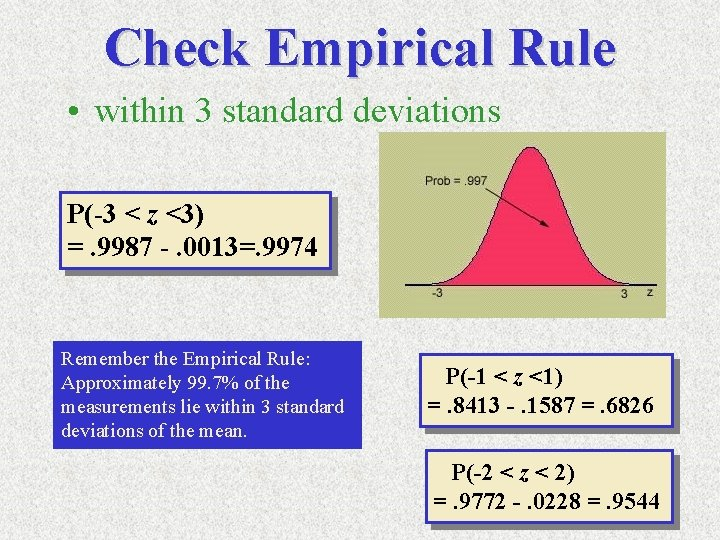Check Empirical Rule • within 3 standard deviations P(-3 < z <3) =. 9987 -. 0013=. 9974 Remember the Empirical Rule: Approximately 99. 7% of the measurements lie within 3 standard deviations of the mean. P(-1 < z <1) =. 8413 -. 1587 =. 6826 P(-2 < z < 2) =. 9772 -. 0228 =. 9544z value with more than two decimals P( z < 1. 643) = P( z < 1. 64) =. 9495 P( z < 1. 6474) = P( z < 1. 65) =. 9505 P( z < 1. 645) ? 1. 1. 645 is halfway of 1. 64 and 1. 65 2. Look for areas of 1. 64 and 1. 65 in Table 3. 3. Since the value 1. 645 is halfway between 1. 64 and 1. 65, we average areas. 9495 and. 9505. 4. P( z 1. 645) = (. 9495+. 9505)/2 =. 9500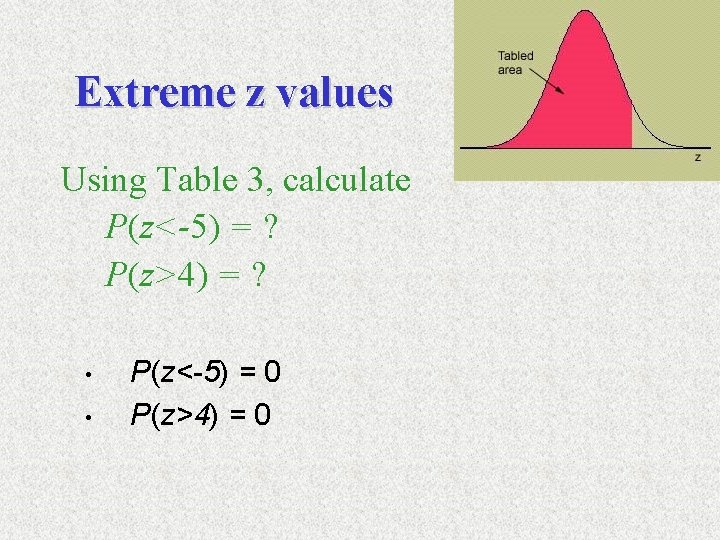Extreme z values Using Table 3, calculate P(z<-5) = ? P(z>4) = ? • • P(z<-5) = 0 P(z>4) = 0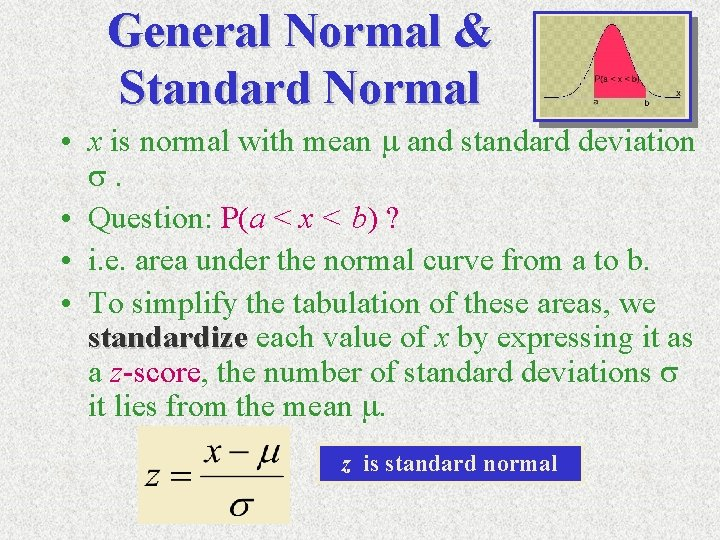General Normal & Standard Normal • x is normal with mean m and standard deviation s. • Question: P(a < x < b) ? • i. e. area under the normal curve from a to b. • To simplify the tabulation of these areas, we standardize each value of x by expressing it as a z-score, the number of standard deviations s it lies from the mean m. z is standard normal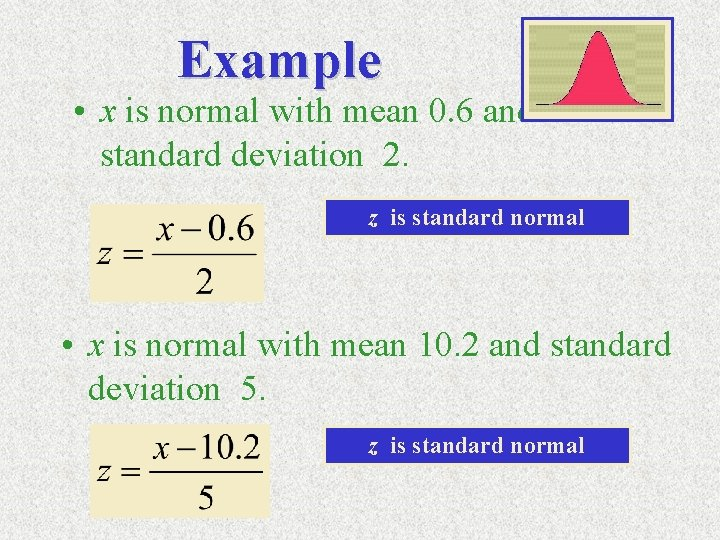Example • x is normal with mean 0. 6 and standard deviation 2. z is standard normal • x is normal with mean 10. 2 and standard deviation 5. z is standard normal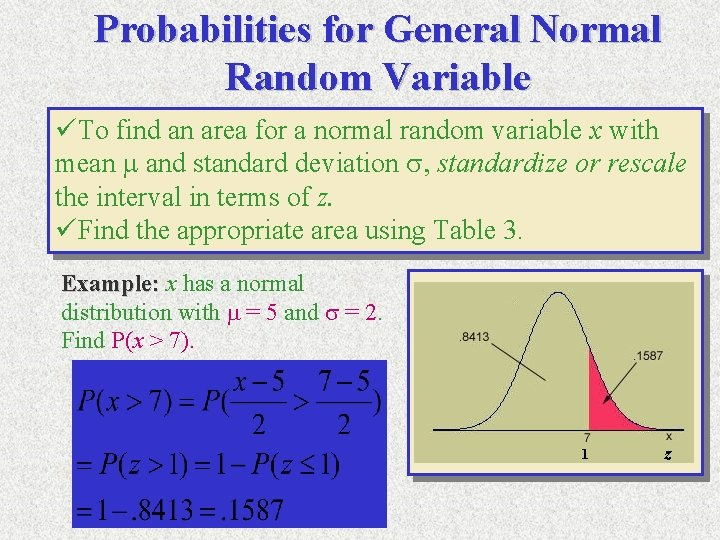Probabilities for General Normal Random Variable üTo find an area for a normal random variable x with mean m and standard deviation s, standardize or rescale the interval in terms of z. üFind the appropriate area using Table 3. Example: x has a normal distribution with m = 5 and s = 2. Find P(x > 7). 1 z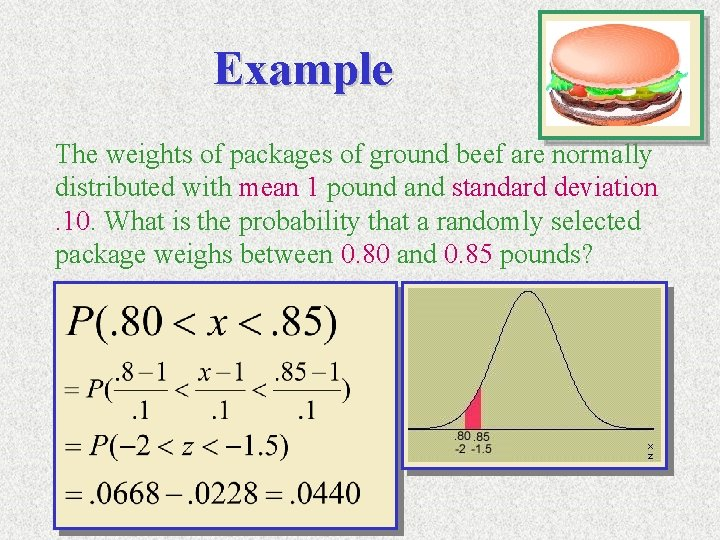Example The weights of packages of ground beef are normally distributed with mean 1 pound and standard deviation. 10. What is the probability that a randomly selected package weighs between 0. 80 and 0. 85 pounds?Area under General Normal Curve Studies show that gasoline use for compact cars sold in U. S. is normally distributed, with a mean of 25. 5 mpg and a standard deviation of 4. 5 mpg. What is the percentage of compacts get 30 mpg or more? 15. 87% of compacts get 30 mpg or more using Table 3Using Table 3 üTo find an area to the left of a z-value, find the area directly from the table. e. g. P( z < 1. 36) üTo find an area to the right of a z-value, find the area in Table 3 and subtract from 1. or find the area with respect to the negative of the z-value; e. g. P( z >1. 36) = 1 -P( z <1. 36), P( z>1. 36) = P( z< -1. 36) üTo find the area between two values of z, find the two areas in Table 3, and subtract. e. g. P( -1. 20 < z < 1. 36 ) üTo find an area for a normal random variable x with mean m and standard deviation s, standardize or rescale the interval in terms of z. üFind the appropriate area using Table 3.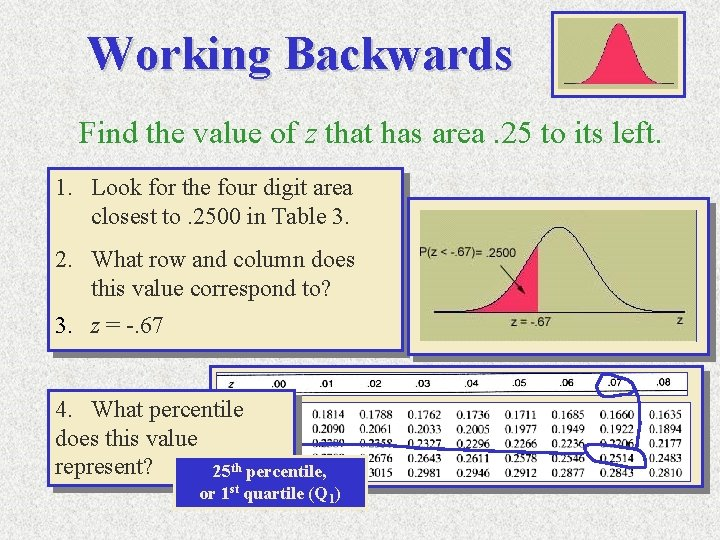Working Backwards Find the value of z that has area. 25 to its left. 1. Look for the four digit area closest to. 2500 in Table 3. 2. What row and column does this value correspond to? 3. z = -. 67 4. What percentile does this value represent? 25 th percentile, or 1 st quartile (Q 1)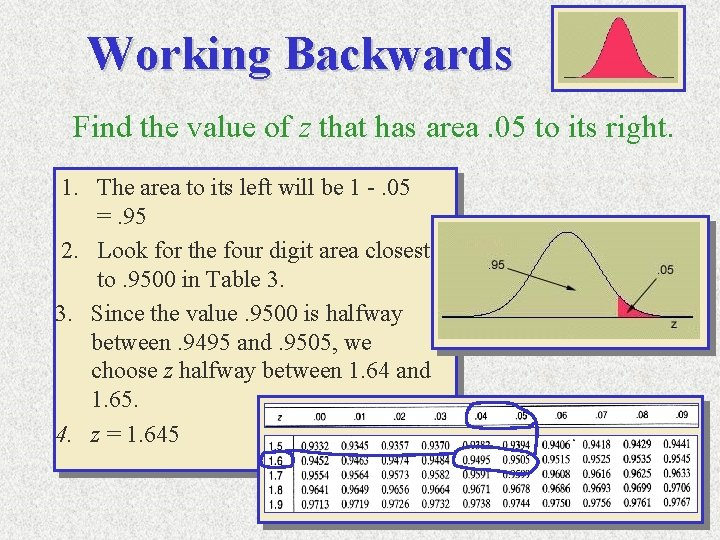Working Backwards Find the value of z that has area. 05 to its right. 1. The area to its left will be 1 -. 05 =. 95 2. Look for the four digit area closest to. 9500 in Table 3. 3. Since the value. 9500 is halfway between. 9495 and. 9505, we choose z halfway between 1. 64 and 1. 65. 4. z = 1. 645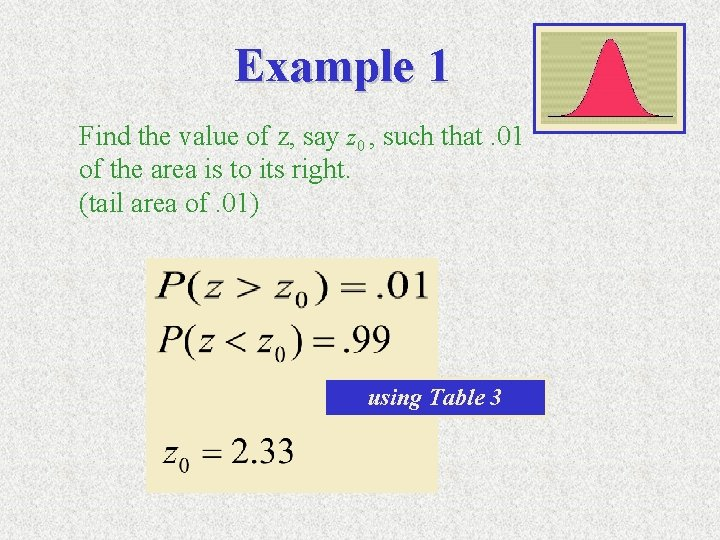Example 1 Find the value of z, say z 0 , such that. 01 of the area is to its right. (tail area of. 01) using Table 3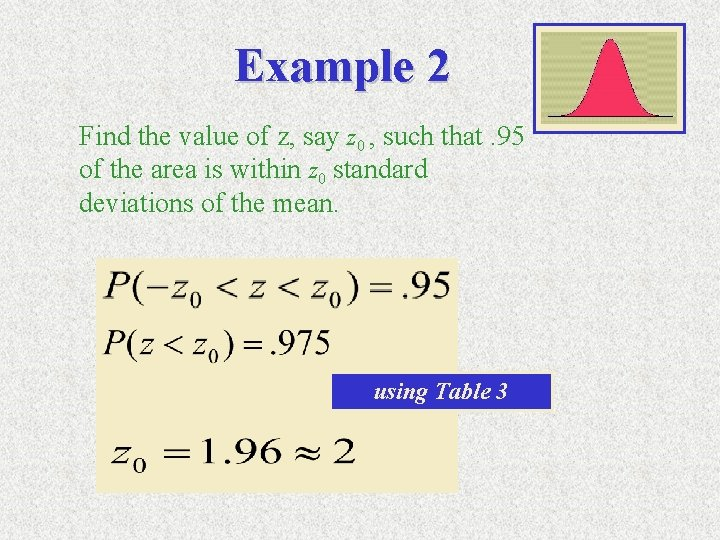Example 2 Find the value of z, say z 0 , such that. 95 of the area is within z 0 standard deviations of the mean. using Table 3Example 3 The weights of packages of ground beef are normally distributed with mean 1 pound and standard deviation. 10. What is the weight of a package such that only 1% of all packages exceed this weight? using Table 3 99 th percentileExercise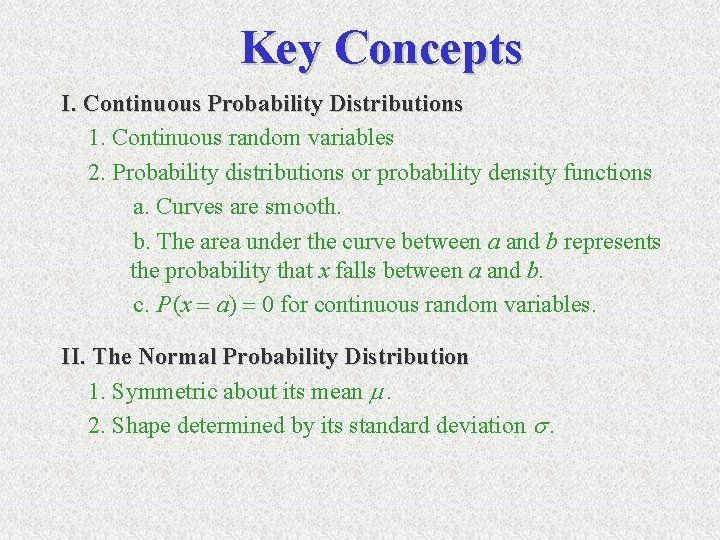Key Concepts I. Continuous Probability Distributions 1. Continuous random variables 2. Probability distributions or probability density functions a. Curves are smooth. b. The area under the curve between a and b represents the probability that x falls between a and b. c. P (x = a) = 0 for continuous random variables. II. The Normal Probability Distribution 1. Symmetric about its mean m. 2. Shape determined by its standard deviation s.Key Concepts III. The Standard Normal Distribution 1. The normal random variable z has mean 0 and standard deviation 1. 2. Any normal random variable x can be transformed to a standard normal random variable using 3. Convert necessary values of x to z. 4. Use Table 3 in Appendix I to compute standard normal probabilities. 5. Several important z-values have tail areas as follows: Tail Area: . 005 . 01 . 025 . 05 . 10 z-Value: 2. 58 2. 33 1. 96 1. 645 1. 28